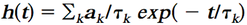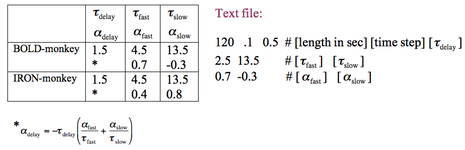## IRF (or HRF)

A hemodynamic response function (HRF) is just an impulse response function (IRF) that models the response of the blood supply in relation to the presumed neural response. A common form of this IRF is the gamma-variate function (e.g., Boyton 1996), or sums of several gamma-variate functions.  Instead, this GLM implementation instead uses sums of exponential functions:There is no particular advantage to either type of IRF form, although with exponentials, the dominant time constants can be more easily viewed from the data.  Convolution of an exponential (exp(-t/tau)) with a step function yields another exponential (1-exp(-t/tau)). The two dominant time constants in BOLD signal are the initial positive response (for "activation" assuming a square neural response) and a post-stimulus undershoot. The two dominant time constants for the IRON response are a rapid and a slow component of increasing CBV. If one only used long block stimuli, these two time constants ("fast" and "slow") would be sufficient. However, very rapid sampling will reveal that another time constant, one that resolves rapidly and functions sort of like a delay, is needed to model the initial rise of the leading edge of the response. Additionally the 3rd time constant is required to form a "proper" IRF with h(0)=0.

In this GLM, the IRF can be specified using built-in functions or by using a text file. In the GLM control file, the keyword "IRF" specifies the type. Recognized arguments are BOLD-monkey, IRON-monkey, BOLD-rat, and IRON-rat. Any other argument is assumed to be a text file.  E.g., "IRF IRON-monkey" is a built-in function, and "IRF my-irf.dat" is a text file.   The form of two built-in functions is shown below, along with a text file that would be roughly appropriate for BOLD signal in the rat.If an IRF is specified in the GLM, then all events except table events are convolved with the IRF in order to form the regressors that are included in the design matrix. Users may wish to convolve some table events but not others (see sidebar). For instance, motion-correction parameters typically would not be convolved, but eye traces, which invoke brain activity, presumably would be convolved.

Joseph B. Mandeville, Athinoula A. Martinos Center for Biomedical Imaging at MGH/MIT/Harvard